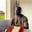Related Tags

algorithms
array
xor
communitycreator

# Find the integer that appears once in an arrayBenjamin Chibuzor-Orie

Problem: Given a non-empty array of integers, where every element appears twice except for one, find that single one (assume that no other integer occurs once).There are several approaches to solving this problem:

• One is to pick each element and check if it occurs twice by sweeping through the array. The problem with this solution is that it has a quadratic time complexity and wouldn’t play well with arrays containing a lot of items.

• A better solution is to sweep through the array once, store each element, and its count in a hash table. Then, simply check the hash table for the item with the lowest count. Now this solution has a linear time complexity, but the problem is that we need extra memory to store our hash table. What if we were memory constrained?

## Optimal Solution

The best solution to this problem is XOR. The XOR operator, which is bundled into almost every programming language on the planet, is one of the most underrated and underused operators (the family of bitwise operators in general), but it’s the only tool that can provide us with a memory-efficient solution to this problem. Bitwise operators convert their operands to their binary form and operate on each corresponding bit in both operands.

So, if you do 5 ^ 7 (assuming ^ is the XOR operator), what happens underneath is:

5 => 101
7 => 111
5 ^ 7 => 1^1 0^1 1^1


The truth table of the XOR table is shown below:

A B X
0 0 0
0 1 1
1 0 1
1 1 0

So the result of 5 ^ 7 is 010 or 2 in decimal. It’s that simple.

The concept that enables us to solve this problem is the fact that when you XOR two equal numbers, the result is zero, and when you XOR any number with zero, the result is that number.

So in an array like this,

{1, 2, 1, 3, 4, 3, 2}


one by one we progressively XOR all the elements of the array.

1 ^ 2 = 3, then
3 ^ 1 = 2, then
2 ^ 3 = 1, then
1 ^ 4 = 5, then
5 ^ 3 = 6, then
6 ^ 2 = 4.


As you can see, 4 happens to be the only element that occurs once in the array. The idea is that duplicates will resolve to zero, in the long run,​ leaving out unique elements (4 in this case).

## Code

Below is a C++ code block that describes the solution:

#include <iostream>
using namespace std;

int main() {
int nums[] = {1, 2, 1, 3, 4, 3, 2};
for (int i = 1; i < 7; i ++){
result ^= nums[i];
}
cout << result << endl;
}

RELATED TAGS

algorithms
array
xor
communitycreator

CONTRIBUTORBenjamin Chibuzor-Orie
RELATED COURSES

View all Courses

Keep Exploring

Learn in-demand tech skills in half the time